# Alpha Decay

(redirected from Alpha-decay)
Also found in: Dictionary, Thesaurus, Medical.

## alpha decay

[′al·fə di′kā]
(nuclear physics)
A radioactive transformation in which an alpha particle is emitted by a nuclide.
McGraw-Hill Dictionary of Scientific & Technical Terms, 6E, Copyright © 2003 by The McGraw-Hill Companies, Inc.
The following article is from The Great Soviet Encyclopedia (1979). It might be outdated or ideologically biased.

## Alpha Decay

(α-decay), the emission of alpha particles by atomic nuclei in the process of spontaneous radioactive decay. In the alpha decay of a parent radioactive nucleus with atomic number Z and mass number A an alpha particle is emitted—that is, a helium nucleus 4He—as a result of which a product nucleus is formed with atomic number Z - 2and mass number A - 4. For example, Ra, in emitting an alpha particle, becomes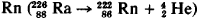About 200 alpha-radioactive nuclei are known to exist (1968); a large percentage of them are heavier than Pb (Z > 82). There are also a number of them among the neutron-deficient nuclei in the category Z < 82—for example, 14662Sm. The energy of alpha decay for all known alpha-radioactive isotopes lies in an interval from 3 to 9 million electron volts; (MeV; see Figure 1). The half-lives of alpha-radioactive nuclei vary over an enormous interval of values—for example, from 3 x 10-7 sec for 212Po to 5 ξ 1015 years for 142Ce. Experimental detection of alpha-active nuclei with mass number A < 200 is hampered by enormous lifetimes characteristic of nuclei with small alpha-decay energies.

Alpha decay of nuclei can occur with the formation of a product nucleus not only in the ground state but also in excited states. For alpha decay of nuclei having an even number of neutrons and protons (even-even nuclei) the most probable formation is a product nucleus in the ground state. In the case of alpha decay of nuclei with an odd number of protons or neutrons (odd-even nuclei) the product nucleus is mainly formed not in the ground state (transitions with maximum alpha-particle energies), but in an excited state with the same spin value as in the ground state of the parent nucleus (see Figure 2). If the fine structure of the spectra of alpha particles is known, the energy of the excited states of the product nucleus may be calculated.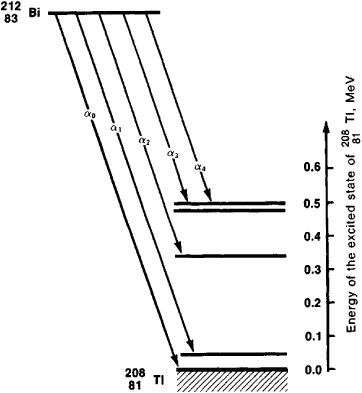Figure 2.

Note to Figure 2.: Energy diagram of alpha decay of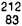Bi with the formation of a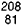Tǀ nucleus in the ground (αO) and excited (α1, α2, α3, α4) states

All methods of describing alpha decay are based on differentiation of the process into two stages: (1) formation of an alpha particle within the nucleus (with some probability) and (2) penetration by the alpha particle of a potential barrier which is formed by the joint action of nuclear forces that are large in magnitude and act at small distances— tending to retain the alpha particle within the nucleus—and a Coulomb (electrostatic) interaction of the alpha particle with the remaining portion of the nucleus (see Figure 3).

The total (potential and kinetic) energy of an alpha particle in different nuclei can be taken to be negative as well as positive (with increased nuclear charge) in value. In the latter case alpha decay is permitted in terms of energy. In Figure 3 the heavy line represents the potential energy of an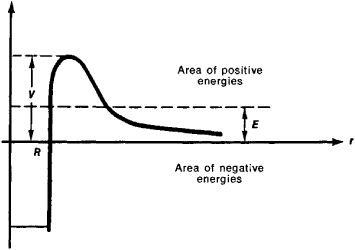Figure 3. Potential energy of interaction of an alpha particle with the product nucleus: V is the kinetic energy of :he escaping alpha particle, r is the distance of the alpha particle from the center of the nucleus, and R is the radius of the nucleus

alpha particle in the nucleus. The kinetic energy of the emitted alpha particle is equal to the energy corresponding to the difference between the mass of the radioactive nucleus and the sum of the masses of the alpha particle and the product nucleus. Although the total energy of the alpha particle is substantially less than the height V of the potential barrier, penetration of the potential barrier by the alpha particle is possible according to the laws of quantum mechanics; it occurs by means of the tunnel effect. The probability λ of alpha decay is approximately described by the formula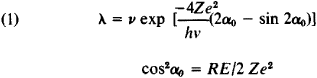Here R is the effective radius of the nucleus, ν is the velocity of the alpha particle in its flight out of the nucleus, e is the charge of an electron, and h is Planck’s constant. Formula (1) leads to the Geiger-Nuttall relation, according to which the half-lives T1 and T2 of two alpha-active nuclei and the kinetic energies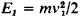and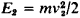of alpha particles emitted by them are bound by the relation

(2) In (T1/T2 ≈ (√E1/E2 - 1)

Formula (1) stipulates the strict dependence of the probability of alpha decay on energy. For example, changing the energy E of the alpha particles by 10 percent leads to a change in λ by a factor of approximately 3.

The factor ν in formula (1) is connected with the probability of formation of an alpha particle on the surface of the nucleus. Originally, in the theory of G. Gamow, the factor was assumed to be equal to v/2R—that is, equal to the frequency with which the alpha particle strikes the “border” of the nucleus. Later, L. D. Landau proposed the expression ν = D/2h, where D is the average distance between levels of the alpha particle in the field of the nucleus. Contemporary theories of alpha decay connect the value ν with the so-called reduced width of alpha decay, which is computed in concrete nuclear models. Calculation of the probability of alpha decay according to formula (1) with the formation of even-even nuclei in the ground state usually leads to results that are underestimated by a factor of 5 to 1,500 in comparison with experimental data; for alpha decay with formation of nuclei in excited states, to overestimated values. Since the probability of alpha decay depends on the dimensions of the nucleus, alpha decay can be used for rough determination of the dimensions of heavy nuclei. The study of alpha decay in nuclei in excited states facilitates research on nuclei with small values of Z (for example, the nuclei of many rare earth elements), for which alpha decay in an unexcited state is not observed. Such excited nuclear states are formed by capturing slow neutrons.

Many basic notions about atomic and nuclear physics owe their very origins to the study of alpha decay. The theory of alpha decay, proposed in 1928 by G. Gamow and independently by R. Gurney and E. Condon, became the first application of quantum mechanics to nuclear processes.

### REFERENCES

Gordanskii, V. I., and E. M. Leikin. Prevrashcheniia atomnykh iader. Moscow, 1958.
Rasmussen, J. “Al’fa-raspad.” In Al’fa-l beta-l i gammaspektroskopiia, issue 2. Edited by K. Siegbahn. Moscow, 1969. Page 137. (Translated from English.)
Popov, I. P. “Alfa-raspad vozbuzhdennykh sostoianii iader, obrazuiushchikhsia posle zakhvata medlennykh neitronov.” In Materialy tret’ei zimnei shkoly po teorii iadra i fizike vysokikh energii. Leningrad, 1968. Page 128. (Published by the A. F. Ioffe Leningrad Physics and Engineering Institute.) [1–1431–6; updated]